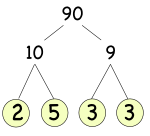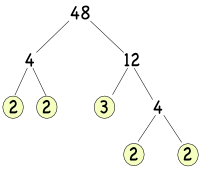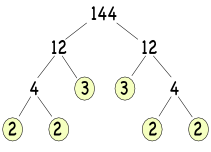Prime Factorisation

## Prime Factorisation

Finding the factors of a number is called factorising. In addition to the numbers 1 and itself, factors of a number can often be factorised themselves.

Any number can be factorised into a set of prime numbers: this is known as the Product of the Prime Factors, or the Product of its Primes (Product is another word for multiplication). The number 24

• can be written as 4 x 6,

• which can then be written as (2 x 2) x (2 x 3).

The Product of the Prime Factors for 24 is 23 x 3.

A Prime Factor Tree can be used to obtain the Product of its Primes for a number. Start with the number. Create a pair of branches: at the end of each branch, enter any pair of factors for the number. Repeat the process for each branch unless it ends in a prime number. Multiply together the primes at the end of each branch.90 = 2 x 5 x 3 x 3, re-arrange to:

90 = 2 x 3 x 3 x 5, re-write as powers

90 = 2 x 32 x 5

Every number greater than 1 is either a prime number or can be represented as the product of its primes. A number will always factorize to the same set of primes, and that set of primes cannot be multiplied together for any different number (the Unique Factorization Theorem).

## Example 1

Write 48 as the product of its primes.Answer: 48 = 24 x 3.

## Example 2

Write 144 as the product of its primes.Answer: 144 = 24 x 32